Next: Schrödinger's Equation Up: Wave-Particle Duality Previous: Evolution of Wave Packets

# Heisenberg's Uncertainty Principle

According to the analysis contained in the previous two sections, a particle wave packet which is initially localized in-space with characteristic width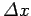is also localized in-space with characteristic width. However, as time progresses, the width of the wave packet in-space increases, whilst that of the wave packet in-space stays the same. [After all, our previous analysis obtained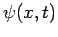from Eq. (97), but assumed that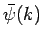was given by Eq. (93) at all times.] Hence, in general, we can say that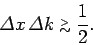(118)

Furthermore, we can think ofandas characterizing our uncertainty regarding the values of the particle's position and wavenumber, respectively.

Now, a measurement of a particle's wavenumber,, is equivalent to a measurement of its momentum,, since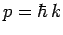. Hence, an uncertainty inof ordertranslates to an uncertainty inof order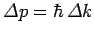. It follows from the above inequality that(119)

This is the famous Heisenberg uncertainty principle, first proposed by Werner Heisenberg in 1927. According to this principle, it is impossible to simultaneously measure the position and momentum of a particle (exactly). Indeed, a good knowledge of the particle's position implies a poor knowledge of its momentum, and vice versa. Note that the uncertainty principle is a direct consequence of representing particles as waves.

It can be seen from Eqs. (79), (105), and (112) that at largea particle wavefunction of original width(at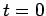) spreads out such that its spatial extent becomes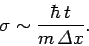(120)

It is easily demonstrated that this spreading is a consequence of the uncertainty principle. Since the initial uncertainty in the particle's position is, it follows that the uncertainty in its momentum is of order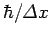. This translates to an uncertainty in velocity of. Thus, if we imagine that parts of the wavefunction propagate at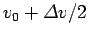, and others at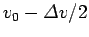, whereis the mean propagation velocity, then the wavefunction will spread as time progresses. Indeed, at largewe expect the width of the wavefunction to be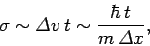(121)

which is identical to Eq. (120). Evidently, the spreading of a particle wavefunction must be interpreted as an increase in our uncertainty regarding the particle's position, rather than an increase in the spatial extent of the particle itself.Figure 8 illustrates a famous thought experiment known as Heisenberg's microscope. Suppose that we try to image an electron using a simple optical system in which the objective lens is of diameter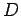and focal-length. (In practice, this would only be possible using extremely short wavelength light.) It is a well-known result in optics that such a system has a minimum angular resolving power of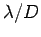, where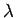is the wavelength of the light illuminating the electron. If the electron is placed at the focus of the lens, which is where the minimum resolving power is achieved, then this translates to a uncertainty in the electron's transverse position of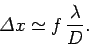(122)

However,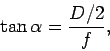(123)

where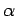is the half-angle subtended by the lens at the electron. Assuming thatis small, we can write(124)

so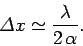(125)

It follows that we can reduce the uncertainty in the electron's position by minimizing the ratio: i.e., by using short wavelength radiation, and a wide-angle lens.

Let us now examine Heisenberg's microscope from a quantum mechanical point of view. According to quantum mechanics, the electron is imaged when it scatters an incoming photon towards the objective lens. Let the wavevector of the incoming photon have the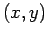components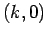. See Fig. 8. If the scattered photon subtends an anglewith the center-line of the optical system, as shown in the figure, then its wavevector is written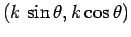. Here, we are ignoring any wavelength shift of the photon on scattering--i.e., the magnitude of the-vector is assumed to be the same before and after scattering. Thus, the change in the-component of the photon's wavevector is. This translates to a change in the photon's-component of momentum of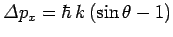. By momentum conservation, the electron's-momentum will change by an equal and opposite amount. However,can range all the way fromto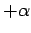, and the scattered photon will still be collected by the imaging system. It follows that the uncertainty in the electron's momentum is(126)

Note that in order to reduce the uncertainty in the momentum we need to maximize the ratio. This is exactly the opposite of what we need to do to reduce the uncertainty in the position. Multiplying the previous two equations, we obtain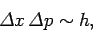(127)

which is essentially the uncertainty principle.

According to Heisenberg's microscope, the uncertainty principle follows from two facts. First, it is impossible to measure any property of a microscopic dynamical system without disturbing the system somewhat. Second, particle and light energy and momentum are quantized. Hence, there is a limit to how small we can make the aforementioned disturbance. Thus, there is an irreducible uncertainty in certain measurements which is a consequence of the act of measurement itself.Next: Schrödinger's Equation Up: Wave-Particle Duality Previous: Evolution of Wave Packets
Richard Fitzpatrick 2010-07-20